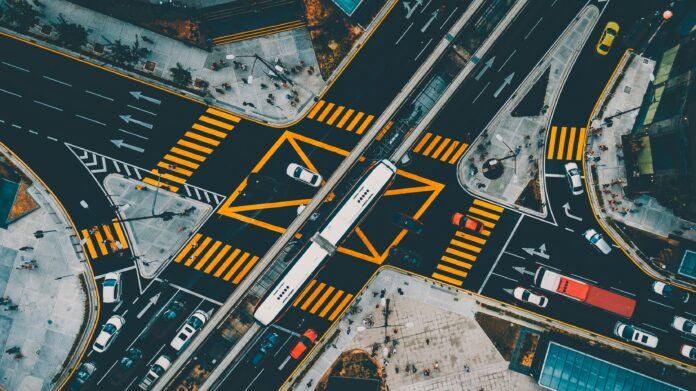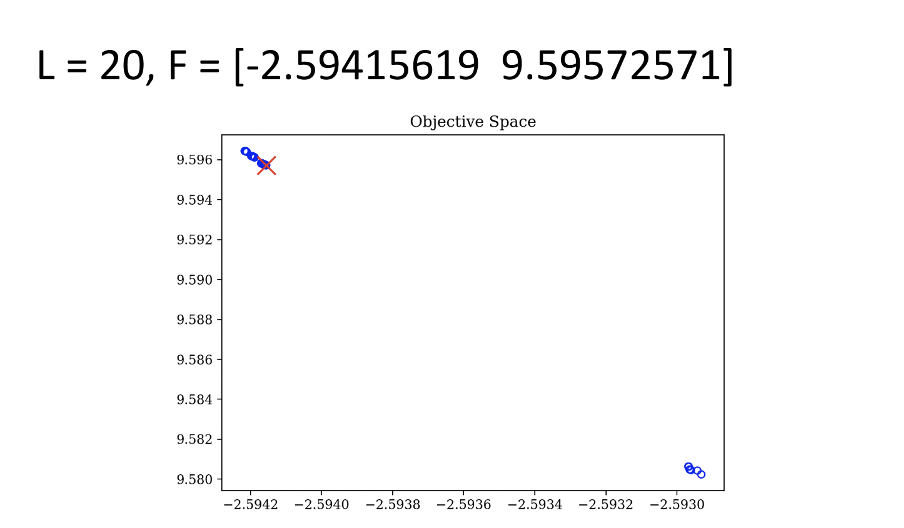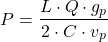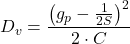# Enhancing pedestrian and Vehicle Flow in One-Way Roads by Optimizing Crosswalk Size with Improved NSGA-II

0
163## Abstract

Finding the optimal size of the crosswalk is a crucial part of maximizing the efficiency of the traffic flow and increasing both pedestrians and drivers’ safety. In this study, an algorithm to produce an optimal solution to minimize vehicle delay and maximize the volume of pedestrians by varying the length of a one-way road crosswalk was developed. Through this research, a simplified model of a one-way crosswalk was selected to focus on the size of the crosswalk primarily. To find an optimal solution, the Non-Dominated Sorting Genetic Algorithm II (NSGA-II) was selected in this research to solve a multiple-objective problem. The results showed similar outcomes for the ratio between vehicle delay and the volume of pedestrians as a function of the crosswalk length that was tested. The reason for this result could be because of the simplified model used to represent this problem. Future work should further investigate the relationship of crosswalk length to vehicle delay and pedestrian volume under more dynamic conditions.

## Introduction

As technology is developing more quickly than at any other time in the past, society is becoming more complex. When considering ways to minimize these complexities, individuals may think about the concept of efficiency throughout the world. Optimization is the process of finding efficient solutions to increasingly complex societal problems, and it is used across a wide variety of fields, such as traffic flows, business operations, logistics, and supply chains. The process of optimizing a system involves identifying an issue, formulating a mathematical representation of the situation, and either minimizing or maximizing the objective function to solve for the most efficient solution, should it exist.  In this paper, we seek to apply optimization to concepts within the field of traffic and pedestrian operations. The focus of this research is investigating how varying the size of the crosswalk located on a one-way road can be optimized for the overall traffic flow, including both pedestrian and vehicle flow. Considering that the given problem is on a one-way road, the size of the crosswalk is a  critical aspect of the efficiency of the overall traffic flow.

As modern society has become more complex, there are many vehicles on the roadway. Efficient traffic flow has increased in criticality since the amount of traffic has increased on the road. Inefficiency can be caused by a lack of efficient time management at traffic lights. If the traffic flow isn’t running efficiently, traffic jams along the roads will occur, which will lead to local and national economic loss1. Inefficiency in traffic flow can also lead to causing safety problems. If traffic flows aren’t arranged well, traffic accidents will happen frequently, causing a safety threat. Inefficient traffic flow can lead to car-to-car accidents, car-to-human accidents, or even human-to-human accidents.

Many previous works have been published investigating the optimization of traffic patterns and efficient crosswalks. A paper  by Chunhui Yu et al.2 focused on maximizing the vehicle bandwidth and minimizing the pedestrian’s delay to focus on the optimization of a crosswalk with midblock. The optimization model in this previous research was run under the Non-Dominated Sorting Genetic Algorithm II (NSGA-II) and focused on the optimal location of the midblock and the proper signal for crosswalks. This research was able to discover the optimal combination between applying midblock and signal timing for each single midblock on a crosswalk and two midblocks on a crosswalk. The paper by Wanjing Ma et al.3 focused on optimizing the crosswalk shape such as the exclusive pedestrian phase (EPP) and conventional two-way crossing (TWC) and the signal timing at the crosswalk. Those factors eventually come together to optimize vehicular traffic and pedestrian movements. This research was able to discover the optimal combination between types of phase patterns of crosswalks and the signal timing while considering vehicular demand, pedestrian demand, proportion of turning movements, and proportion of diagonal crossing pedestrians. These two previous papers developed successful optimal solutions, which are currently applied in China. These papers focused on more specific events, such as having midblock and diagonal crossing. In this research, optimal solutions for more general crosswalks are discovered.

However, some researches related to crosswalk optimization also tend to use other methods besides NSGA-II. A paper by Lili Lu et al.4 focused on studying a new approach to specify the width of crosswalks by developing the model which incorporates social forces (SFCA). Through this model, they were able to determine recommended maximum and minimum width of crosswalks under different pedestrian volumes. A paper by Wael K. M. Alhajyaseen el al.5 focused on discovering the system that geometry and operational system would be simultaneously optimized. The research aimed to propose new criteria for crosswalk width, which considers pedestrian demand and  its characteristics. These papers focused on social interactions between the pedestrians on the crosswalk in order to optimize the crosswalk width.

First two research papers selected the Non-Dominated Sorting Genetic Algorithm II (NSGA-II) to check their model efficiency2,3. NSGA-II is an algorithm for solving multi-objective functions, and according to the previous paper by Wanjing Ma et al.3, “It is efficient for solving multi-objective signaling problems through NSGA-II.” In this paper, since coding process was used to solve for an algorithm which had two objective functions, NSGA-II was selected which is able to solve for multi-objective signaling problems. SFCA which was used in one of the previous papers would not fit since SFCA is focusing on the model while objective functions requires an algorithm to result pareto-front. In this paper, the Non-Dominated Sorting Genetic Algorithm II, NSGA-II, will be run through Python. Then, the data set will be analyzed, and the problem will be solved through an optimization process.

In this paper, we seek to apply optimization to concepts within the field of traffic and pedestrian operations. The focus of this research is investigating how varying the size of the crosswalk located on a one-way road can be optimized for the overall traffic flow, including both pedestrian and vehicle flow. Considering that the given problem is on a one-way road, the size of the crosswalk is a  critical aspect of the efficiency of the overall traffic flow. NSGA – II will be utilized throughout the reesarch to find optimal solution.

## Results

This work developed a mathematical representation of the problem space that included the length in the model formulation. The problem was solved using NSGA-II in Python. The custom Python script resulted in a graph of the Pareto front to show optimal solutions of reducing the vehicle delay on the y-axis and maximizing the volume of pedestrians on the x-axis. The volume of the pedestrian is in the negative value because of the process of maximizing the number of pedestrians, so the absolute value of the numbers on the x-axis would be the actual volume of pedestrians. The importance between two factors, vehicle delay and the volume of pedestrians, were set equally to 50%, meaning both factors were treated equally in terms of their importance.

Figure 1 displays that when the length was set as 20m, it was the most optimal situation when vehicles were delayed for 9.5957 seconds, and pedestrians were in the size of 2.5942.Figure 2 displays that when the length was set as 40m, it was the most optimal situation when vehicles were delayed for 11.9553 seconds, and pedestrians were in the size of 4.4591.

Figure 3 displays that when the length was set as 60m, it was the most optimal situation when vehicles were delayed for 15.9578 seconds, and pedestrians were in the size of 7.7305.

## Discussion

The results show that the crosswalk size, more specifically, the length of the crosswalk, is crucial for varying the volume of pedestrians and the vehicle delay. As in the figures above, the range of each result for x and y axis is mainly in the range of one integer number, which tells that length is a crucial value to manipulate the optimal result.

The premise of this paper is that varying the size of the crosswalk, which is the length of a one-way road in this case, would be optimized by minimizing the delay for vehicles and maximizing the volume of pedestrians crossing the crosswalk. By doing so, vehicle delay can be reduced, and a greater number of pedestrians will be able to cross the one-way road. Therefore, the result of each length of 20m, 40m, and 60m shown in the figures above visualize the optimized ratio between the seconds for vehicle delay and the number of pedestrians crossing the crosswalk.

NSGA-II was run through Python in this research to optimize the vehicle delay and the volume of pedestrians depending on the size of the crosswalk on the one-way road. A mathematical formulation to relate the length of the crosswalk to the vehicle delay and the volume of pedestrians was created. The range of results shown on each axis is negligible, which tells us that the length of the crosswalk is a dominant variable determining an optimal solution, such that the Pareto front covers a very small subset of optimal solutions.

For the crosswalk with a length of 20m, the most optimized ratio between seconds for vehicle delay and the number of pedestrians was 9.6 seconds to 2.6 people. For the crosswalk with a length of 40m, the most optimized ratio between seconds for vehicle delay and the number of pedestrians was 12.0 seconds to 4.6 people. For the crosswalk with a length of 60m, the most optimized ratio between seconds for vehicle delay and the number of pedestrians was 16.0 seconds to 7.7 people. These results from the algorithm provided the ideal ratio between the seconds for vehicle delay and the number of pedestrians. When constructing the crosswalk of each length of 20m, 40m, and 60m on a one-way road, it would be strongly suggested to keep the ratio of roughly 3:1 for vehicle delay to the volume of pedestrians for less than 40m length crosswalks on a one-way road.

In this research, for simplicity, other factors such as the width of the crosswalk, cars turning right or left, different types of crosswalks, and the presence of the mid-block on the road were neglected in this paper. However, future work will include the factors omitted for problem simplification.  As more factors are considered within the problem formulation, this work could produce more optimized results and help the local community to design their crosswalks more efficiently. Also, those factors are important since they are more directly connected to the efficiency and the safety of traffic, which would eventually affect the local economy6. For example, according to the paper by Ian Thomson and Alberto Bull, traffic congestion will lead to increased bus fares6. According to the paper, the largest cities in Brazil estimated that congestion increased bus operating costs by up to 16%6. As this case study shows, traffic flow impacts the local economy in a variety of ways. Therefore, optimizing crosswalk length to reduce vehicle delay and maximizing pedestrian crossing will be economically beneficial.

## Methods

### Notations and Parameters:

Table 1. Notations and Parameters used in this experiment

### Model Formulation

Table 2. Parameters used in optimal solution functions

### Objective Function (Maximization):### Objective Function (Minimization):Since units of Q and S are in hours, and other parameters’ values are in seconds, the value of Q and S were converted to a representation in seconds.

Python codes to run an NSGA-II algorithm were created by using the Pymoo8  package. With the assistance of the Pymoo8 resource guide, I was able to develop a custom code to assess this problem through NSGA-II9 with Python. Two objective functions were adapted to optimize the size of the one-way crosswalk. After inserting objective functions into the Python code provided by Pymoo8 for the NSGA-II algorithm9, the algorithm was run through Python for analysis, resulting in the figures shown above (Fig. 1, Fig. 2, Fig. 3 in results section). The figures visualize the optimal results for minimizing the vehicle delay time (in seconds) and maximizing the volume of pedestrians that can cross the street. As noted earlier, the importance between two objective functions was set equally so that while running the Python script, the algorithm is able to analyze the results with equal value placed on each objective function.

## Acknowledgments

This research was executed under the Lumiere Education research program. I would like to thank Jacqueline Hannan from the University of Michigan for advising me throughout the paper’s publishing process.

1. M. Sweet. Does traffic congestion slow the economy?. ResearchGate. 26, 391-404 (2011). []
2. C. Yu, W. Ma, X. Yang, Integrated optimization of location and signal timings for midblock pedestrian crosswalk. Wiley Online Library. 50, 552-569 (2015). [] []
3. W. Ma, Y. Liu, K. L. Head, Optimization of pedestrian phase patterns at signalized intersections: a  multi-objective approach. Wiley Online Library. 48, 1138-1152 (2014). [] [] []
4. Lu, A. L., Ren, B. G., Wang, C. W., & Chan, D. C. Y. (2015). Application of SFCA pedestrian simulation model to the signalized crosswalk width design. Transportation Research Part A: Policy and Practice80, 76-89. []
5. Alhajyaseen, W. K., & Nakamura, H. (2012). Design criteria for crosswalk width and position at signalized intersections. Journal of Civil Engineering and Architecture6(6), 844-857. []
6. I. Thomson, A. Bull, Urban traffic congestion: its economic and social causes and consequences. CEPAL REVIEW. 76, 105-116 (2002). [] [] []
7. Ma, Y. Liu, K. L. Head, Optimization of pedestrian phase patterns at signalized intersections: a  multi-objective approach. Wiley Online Library. 48, 1138-1152 (2014). [] [] []
8. J. Blank, K. Deb, Pymoo: multi-objective optimization in python, https://pymoo.org/algorithms/moo/nsga2.html, (2020). [] [] []
9. K. Deb, S. Agrawal, A. Pratap, T. Meyarivan, A fast elitist non-dominated sorting genetic algorithm for multi-objective optimization: NSGA-II. Lecture Notes in Computer Science. 1917, 849-858 (2000). [] []